• 这是一个Range对象基本操作实例，对指定单元格赋值，然后使用弹窗获取值。 代码如下： Sub test1() Worksheets("Sheet1").Range("A5").Value = 22 MsgBox "工作表Sheet1内单元格A5中的值为" _ & ...这是一个Range对象基本操作实例，对指定单元格赋值，然后使用弹窗获取值。

代码如下：

Sub test1()
Worksheets("Sheet1").Range("A5").Value = 22
MsgBox "工作表Sheet1内单元格A5中的值为" _
& Worksheets("Sheet1").Range("A5").Value
End Sub

转载于:https://www.cnblogs.com/OliverQin/p/5753789.html

展开全文• VBA中可以使用数组处理工作表中的数据，是个尽人皆知的秘密了，读取数据加载到数组中也很简单。 工作表中数据如下图所示，需要将B1:G1的内容加载到数组中。这个该如何弄到一个数组中呢，先分别读入数组，然后再使用...

VBA中可以使用数组处理工作表中的数据，是个尽人皆知的秘密了，读取数据加载到数组中也很简单。
工作表中数据如下图所示，需要将B1:G1的内容加载到数组中。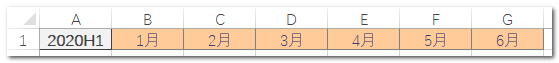哒哒，一行代码瞬间生成一个二维数组，不要问为什么是二维数组？为什么使用方括号？如果真的对这两点有疑问，请自行爬楼翻翻我以前的博文，都有详细讲解。

Sub Demo1()
arr = [B1:G1]
End Sub

可是某些英明（奇葩 ）的领导非要这样的格式，完全不知道这是为了个毛呀！？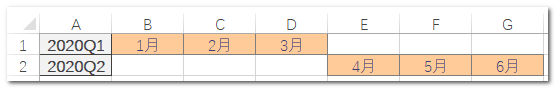这个该如何弄到一个数组中呢，先分别读入数组，然后再使用动态数组就和合并成2x3的数组了。

Sub Demo2()
Dim crr(1 To 2, 1 To 3)
arr = [B1:D1]
brr = [E2:G2]
For i = 1 To 3
crr(1, i) = arr(1, i)
crr(2, i) = brr(1, i)
Next
End Sub

有些不甘心，这么简单的一个事情，要这么多行代码。千万不要忘记，我们是在用Excel中玩儿VBA，Excel中强大的公式可以把这个简化为一行代码就能实现。
啥也别说了，直接上代码。

Sub Demo3()
arr = [Choose({1;2},B1:D1,E2:G2)]
brr = [if({1;0},B1:D1,E2:G2)]
End Sub

【代码解析】
两行代码都可以实现一次性加载到2x3数组中，实现思路是相同的，只是使用的公式不同。
Choose函数根据指定的索引值，从参数串中选择值。此处的参数是两个单元格区域，这样就分两次分别选中了B1:D1和E2:G2单元格区域。
工作表中的If函数将不用解释了，这里需要注意的是，数组{1;0}两个数字的顺序和后面单元格区域的顺序要正确匹配。

看似旁门左道，其实是超级敏捷的实现方式，综合使用Excel的各种技能，才是最高境界。

展开全文数组赋值 VBA 公式
• 在代码窗口，输入CalcCost过程，如下面所示。这个过程基于下述假设来计算购买一个计算器的价钱：计算器的价格为37 美元，销售税为8.5%。代码为： Sub CalcCost() slsPrice = 37 slsTax = 0.085 ...

在代码窗口，输入CalcCost过程，如下面所示。这个过程基于下述假设来计算购买一个计算器的价钱：计算器的价格为37

美元，销售税为8.5%。代码为：

Sub CalcCost()
slsPrice = 37
slsTax = 0.085
Range("A1").Formula = "The cost of calculator"
Range("A4").Formula = "Price"
Range("B4").Formula = slsPrice
Range("A5").Formula = "slsTax"
Range("A6").Formula = "Cost"
Range("B5").Formula = slsPrice * slsTax
Cost = slsPrice + (slsPrice * slsTax)
With Range("B6")
.Formula = Cost
.NumberFormat = "0.00"
End With
strMsg = "The calculator total is " & "$" & Cost & "." Range("A8").Formula = strMsg End Sub 结果显示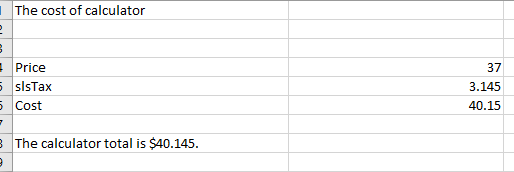解释说明： strMsg = "The calculator total is " & "$" & Cost & "."

1、字符串“The calculator total is ”被引号包括起来了，注意，后面的引号前有个空格。

2、字符&让你将一个字符串附加在另一个字符串或者变量的内容后面

3、在引号里面的美元符合（“$”）用来表明货币类型。因为美元符合是字符，它需要用引号来包括起来 4、字符&必须用于每次你要在前面的字符串后加新信息的时候 5、变量Cost是一个占位符，当过程运行时，计算器的实际价格将显示在这儿 6、字符&可以连接任何字符串 7、句号用引号包括起来。当你需要在句子后面加句号时，如果它是在一个变量后面时，你必须单独再在后面加上它。 如果考虑Cost等值的小数点格式等情况： 可以使用相应的Format命令对相应的对象进行格式要求： Sub CalcCost() slsPrice = 37 slsTax = 0.085 Range("A1").Formula = "The cost of calculator" Range("A4").Formula = "Price" Range("B4").Formula = slsPrice Range("A5").Formula = "slsTax" Range("A6").Formula = "Cost" Range("B5").Formula = slsPrice * slsTax Cost = Format(slsPrice + (slsPrice * slsTax), "0.00") ' Cost = slsPrice + (slsPrice * slsTax) ' With Range("B6") ' .Formula = Cost ' .NumberFormat = "0.00" 'End With Range("B6").Formula = Cost Range("B5").Formula = Format((slsPrice * slsTax), "0.00") strMsg = "The calculator total is " & "$" & Cost & "."
Range("A8").Formula = strMsg
End Sub

税费和Cost都变为2位小数：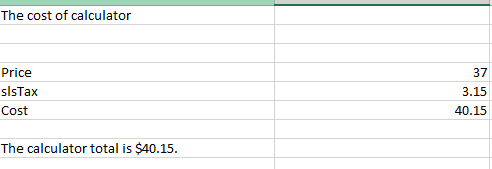展开全文excel vba 程序设计
• 2 如果是，给多个变量赋值，千万别这样写 ：n1 = n2 = n3 这样写，一个变量的赋值都不会对，全是错误的 Sub te1() '坑1， 还有赋值不能多个，只有 private 等范围声明是可以这样的 'ee1 = ee2 = ee3 = 0 '坑？ ...

1连续声明多个变量的作用域，这样写没问题

Private a1, a2, a3, a4a, a50
Private s1, s2, s3

2 如果是，给多个变量赋值，千万别这样写 ： n1 = n2 = n3

这样写，一个变量的赋值都不会对，全是错误的

Sub te1()
'坑1， 还有赋值不能多个，只有 private 等范围声明是可以这样的
'ee1 = ee2 = ee3 = 0 '坑？
n1 = n2 = n3 = 1

Debug.Print "n1=" & n1
Debug.Print "n2=" & n2
Debug.Print "n3=" & n3

h1 = 2
h2 = 2
h3 = 2

Debug.Print "h1=" & h1
Debug.Print "h2=" & h2
Debug.Print "h3=" & h3

End Sub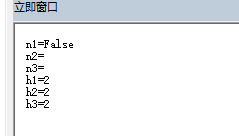3 写个例子试验下

Sub te2()
'重构，重新写

n1 = n2 = n3 = 1
fff1 (n1)
fff1 (n2)
fff1 (n3)

h1 = 2
h2 = 2
h3 = 2
fff1 (h1)
fff1 (h2)
fff1 (h3)

End Sub

Function fff1(a)
Debug.Print "=" & a
End Function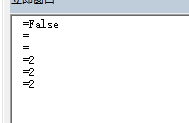展开全文vba
• 以前的博客文章中讲过一些数组的使用技巧，经常会被网友问道如何给数组赋值，本文讲解如何为一维数组赋值（或者说如何创建一维数组）。数组 数组赋值 一维数组 转置
• 在用循环法给给arrnew数组赋值时候， newarr（i，j）=arr（k，j） arr（k，j）有数值，但arrnew=0，实在搞不懂为什么是0，然后即时窗口显示false 被这类问题困扰许久了，烦请各位高手帮忙看看，感激不尽~~~...mysql 开发语言
• Sub aaaa() '行 For i = 1 To Sheet1.Range("A65535").End(xlUp).Row If Sheet1.Cells(i, 1) Then Sheet2.Cells(i, 1) = Sheet1.Cells(i, 1) Else 'asdfasdf ...VBA
• vba给数组赋值

万次阅读 2016-02-24 11:41:05
'向VBA数组中写入数据    '1、按编号(标)写入和读取     Sub t1() '写入一维数组   Dim x As Integer   Dim arr(1 To 10)  arr(2) = 190  arr(10) = 5   End Sub    Sub t2() '向二维数组写入数据和读取 ...
• Sub 属性() ...'VBA对象属性的赋值 Sub 属性赋值() Sheet2.Name = "效率" Sheet2.Range("a1") = "hello vba" End Sub Sub 选择方法() Range("a1:a10")...
• 数组大小不确定，不适合直接给较大的固定长度，直接赋值会出现数组下标越界的错误，该怎么办vba动态数组赋值
• VBA练习Split Excel取值赋值 For循环 数组上限下限LBound UBound
• 了解VBA的变量声明及赋值

千次阅读 2019-03-06 00:21:31
• 俺也不是VBA学员，只得从网上临时学习VBA知识，以解其燃眉之急！现将心得体会与大家分享，虽然不是啥好方法，但是足以应付她的问题了，凡事只可问心无愧，本文绝无炫耀之意；希望对大家有所帮助！注：图片中显示的...
• 不过，实验发现，VBA对变量类型没有进行严格的管控，不管哪种情况，定义的类型和实际使用中赋值类型并没有什么关系，也就是说定义为integer的变量同样赋值字符串，反之也然。 这样的结果往往会在程序中产生歧义，...
• 今天遇到一个问题，结果发现是因为vba中if的判断条件中等号("=")无法完成赋值操作造成的。 　举个栗子： 　 　故应该在if之前就完成必要的赋值操作： 所以，if判断条件里的等号("=")只能用于判断相等，不能...
• 以前的博客文章中讲过一些数组的使用技巧，经常会被网友问道如何给数组赋值，本文讲解如何为二维数组赋值（或者说如何创建二维数组）。VBA 数组 二维数组 赋值 转置
• VBA给合并单元格黏贴赋值

千次阅读 2013-11-06 12:33:49
所以在vba中也一定不能了，其实可以通过一种workaround的方式来间接实现这个需求，实现步骤如下 一、拆分合并的单元格 二、给第一个单元格赋值 三、合并单元格 下面是具体的使用 Sub tttt()  Dim i, g ...vba excel
• VBA常用的系统空值关键字：nothing ,empty ,null 1.1 三者区别 empty 有效的空值，如0 ""，比较常用 null 无效的控制，比如二选一之外的空 nothing 对象变量的空值, 语法是 object is nothing 而不是用 =...
• 使用VBA对指定的单元格赋值并填充颜色 ====================================================== 代码区域 ====================================================== Sub row应用() For Each rw In Rows(&...
• 虽然VBA允许，不对变量赋初值，不定义，直接开始使用。但确实不是什么好习惯 模块开头加上 option explicit 吧， 然后，先定义 x=0， y=1 等等 变量没有初值的情况，在第一次数值运算时 会被默认为0 ，x+1=1 ...
• VBA中对set和dim理解

千次阅读 2020-04-01 00:16:35
Set object = expression 根据前言： 我们给对象赋值是有好处的 比如现在有一个worksheet对象 Dim sht as Worksheet ’创建一个变量sht 他拥有worksheet的属性和方法 for i =2 to 4 set sht = worksheet.add （注意...vba
• <p>factory talk SE软件里怎么写VBA脚本来实现一个报警一天弹框报警的次数，每天只弹一次</p>开发语言 有问必答
• VBA二维数组怎么赋值？

千次阅读 2020-12-22 18:26:51
展开全部可通过 “ = ” ...在VBA中对变量进行赋值可通过 “ = ” 符号或 “ Set ” 关键字进行。通过 “ = ” 符号对变量赋值的语法格式有以下几种。变量名=数据变量1=变量2 运算符 数据变量1=变量2 运算符 变量3...vba二维数组初始化
• VBA复制Excel数据速度过慢解决方法 在使用VBA语句实现对Excel文件自动化处理过程中，当所操作的数据量很大时，会发现程序执行起来很慢，而且Excel文件中行数越多，速度越慢，这时除了改进程序，编写得更加高效方法外...vba excel
• 只是定义了数组，但由于把数组赋值时放到了if语句里，导致可能由于没有符合if语句的情况而没有给数组具体赋值，然后对数组进行其他操作（比如进行数组内数值排序）就会报错。 我想问下，怎么判断通过dim arr()...mysql
• 文章目录概述测试速度的代码测试结果结论 概述 在设置For的开始和结束值时，往往会使用公式，即需要计算才能得到值。但For会不会每次循环都计算一次结束值呢，那岂不是很浪费时间？这就需要来测试一下。......